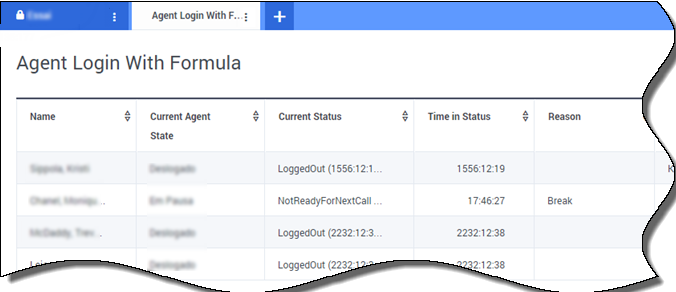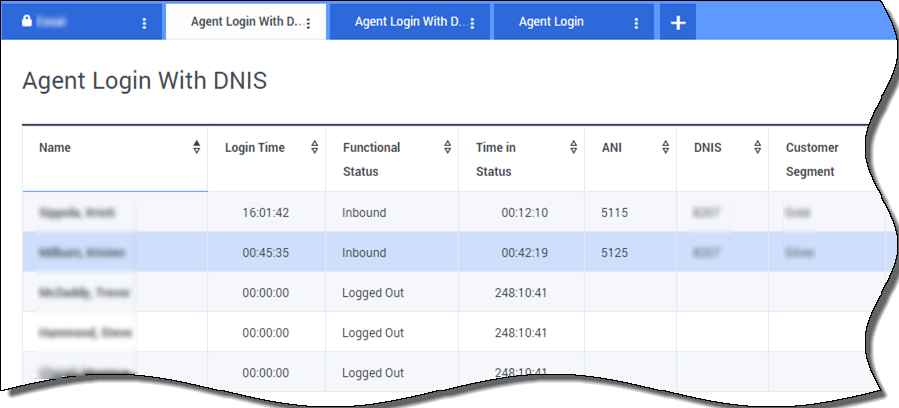# Report Formulas

If you decide that one of your reports needs a different or additional statistic, you can edit the report’s template to make that happen. You can accomplish this by adding a formula to the report template that retrieves the statistic or key performance indicator (KPI) you want.

Since you cannot change the standard templates provided, if you want to change one of the standard reports, just create a clone of the template and make changes in the new template.

Who can create these statistics? If you can create and edit Genesys Pulse templates, you can use formulas.

Important

From the statistic detail pane while editing a widget or template, you can create or customize statistics by creating a formula.

The formula uses a javascript-based syntax, which lets you calculate expressions with values given by other statistic and use functions provided by Genesys for more specific calculations. For example, you can calculate the ratio of the calls abandoned to the calls offered in your queue to measure the percentage of abandoned calls in your queue.

## Display Percentages

Let us say you want to display percentages based on two metrics. Just copy the following example using the statistics you want.

In this example, we want to retrieve the percentage of outbound calls out of the total of both inbound and outbound calls. The formula can access any statistic within a template with the following syntax: Data.Statistic-Alias.Value. The formula must return a valid Result value.

In the following formula, we assume the outbound calls are defined by a statistic alias Outbound and the inbound calls are Inbound.

Formula: Calculate a Percentage

```if ((Data.Outbound.Value + Data.Inbound.Value) != 0)
Result = 100 * Data.Outbound.Value / (Data.Outbound.Value + Data.Inbound.Value);
else Result = 0;```

## Display Agent Status KPIs

Let us say you want to display KPIs for agent status. Just use the Current_Status statistic.### How the Current_Status Statistic is Defined

The Current_Status statistic is defined by Stat Server options properties. The statistic type ExtendedCurrentStatus returns a specific object that can be further analyzed to provide only the Duration of the object.

```[ExtendedCurrentStatus]Category=CurrentStateMainMask=*Objects=AgentSubject=DNAction
```

You can use formulas to find the information you need:

### Show Agent Time in Current State

You can display the agent status duration using the Current_Status statistic.

Formula: Get Status Duration

`Result = G.GetStatusDuration(Data.Current_Status.Value);`

### Show the Reason Code Selected by the Agent

You can display the reason code for the agent status.

Formula: Get Reason Code

`Result = G.GetReasonCodes(Data.Current_Status.Value);`

If you want to display more user data in addition to the Reason Code, you need to enable the Additional Data property (User Data) of the statistic and apply a formula to filter only the Reason Code from the resulting Current_Status, which contains both the User Data and Reason code.

Formula: Filter only Reason Code

```var res = G.GetReasonCodes(Data.Current_Status.Value);
var x = res.split(';');
Result = "";
for (var i = 0; i < x.length; i++) {
var s = x[i];
if (s.indexOf("Break") > -1 ||
s.indexOf("Offline") > -1 ||
s.indexOf("Training") > -1 ) { Result = s; break; }
}```

Formula: Get Reason Code by Media Type (chat in the example below)

```function GetNRCode(state) {
if (state === null || state.type !== "AgentCurrentState")
return null;

var res = "";
var n = state.DNs.length;

if (n > 0) {
for (var i = 0; i < n; ++i) {
var dn = state.DNs[i];

if (dn.DNType === CFGNoDN && dn.DN === "chat") {
var actionsLength = dn.Actions.length;

for (var j = 0; j < actionsLength; j++) {
if (dn.Actions[j].Action === "NotReadyForNextCall" ) {
var userDataLength = dn.Actions[j].Data.UserData.length;

if (userDataLength > 0) {
for (var k = 0; k < userDataLength; k++) {
if (dn.Actions[j].Data.UserData[k].Key === "ReasonCode") {
res = dn.Actions[j].Data.UserData[k].Value;

break;
}
}
}
}
}

break;
}
}
}

return res;
}

Result = GetNRCode(Data.Current_Status.Value);```

Formula: Get Reason Code For Voice

```function GetVR(state) {
if (state === null || state.type !== "AgentCurrentState")
return null;

var res = "";
var n = state.DNs.length;

if (n > 0) {
for (var i = 0; i < n; ++i) {
var dn = state.DNs[i];

if (dn.DNType === 1 && dn.DN !== null) {
var actionsLength = dn.Actions.length;

for (var j = 0; j < actionsLength; j++) {
var userDataLength = dn.Actions[j].Data.UserData.length;

for (var h = 0; h < userDataLength; h++) {
if (dn.Actions[j].Data.UserData[h].Key === "ReasonCode") {
res = dn.Actions[j].Data.UserData[h].Value;

break;
}
}

break;
}
}

break;
}
}
}

return res;
}

Result = GetVR(Data.Current_Status.Value);```
Tip

### Show Current Agent State by Media Type

You can display the current agent state by media type.

Formula - Get agent state by media type

`Result = G.GetAgentNonVoiceStatus(Data.Current_Status.Value, 'email');`

## Display Agent Skills

You can display agent skills using the following formula. The result includes the name and level of each skill the agent has.

```Result = "";
if (Object.Skills != null) {
for (var i = 0; i < Object.Skills.length; i++) {
var skill = Object.Skills[i];
Result += skill.Name + " " + skill.Level +"; ";
}
}```

## Display Interaction Properties

Let us say you want to display interaction properties including flow segmentation, ANI, and DNIS. You can use formulas to find the information you need:### Show the Customer Segment of the Interaction

You can display the customer segment defined by the CustomerSegment key-value pair of the interaction by using the following formula.

Formula: Get Customer Segment

`Result = G.GetCustomerSegment(Data.Current_Status.Value);`

### Show the ANI of the Customer

You can display the ANI of the customer by using the following formula.

Formula: Get ANI

`[Result = G.GetANI(Data.Current_Status.Value);`

### Show the DNIS of the Customer

You can display the DNIS of the customer by using the following formula.

Formula: Get DNIS

`Result = G.GetDNIS(Data.Current_Status.Value);`